# OECD Non-Financial Corporations Debt to Surplus Ratio Analysis 4 - t-test, Wilcoxon rank sum test and correlation test using RUnsplashmartin bennieが撮影した写真

This post is following of above post.

Let's calculate difference between Y2016 and Y2015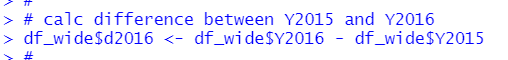Let's see a histogram of d2016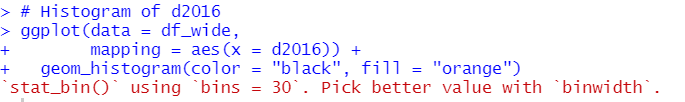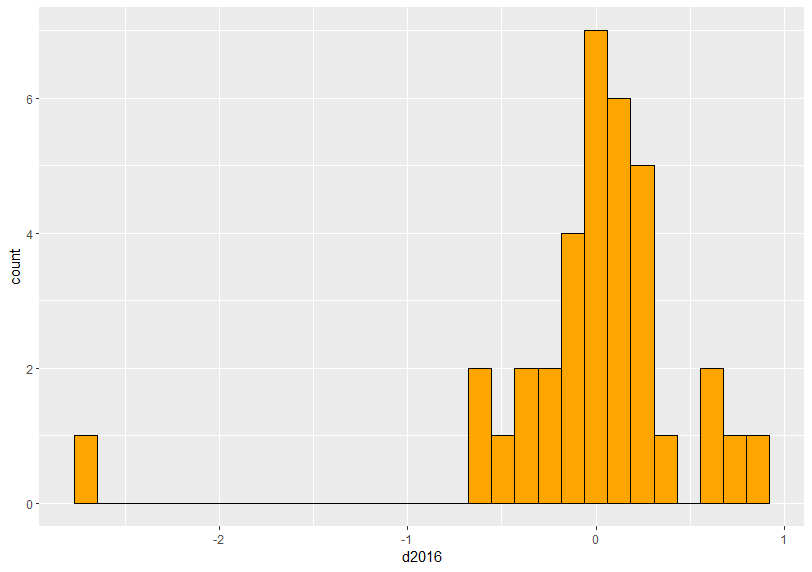Then, let's calculate difference between Y2017 and Y2016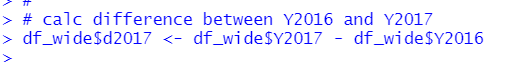Let's make a histogram of d2017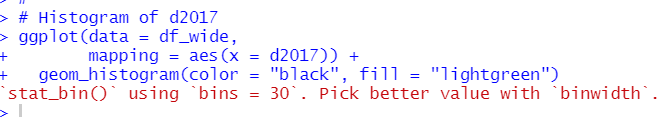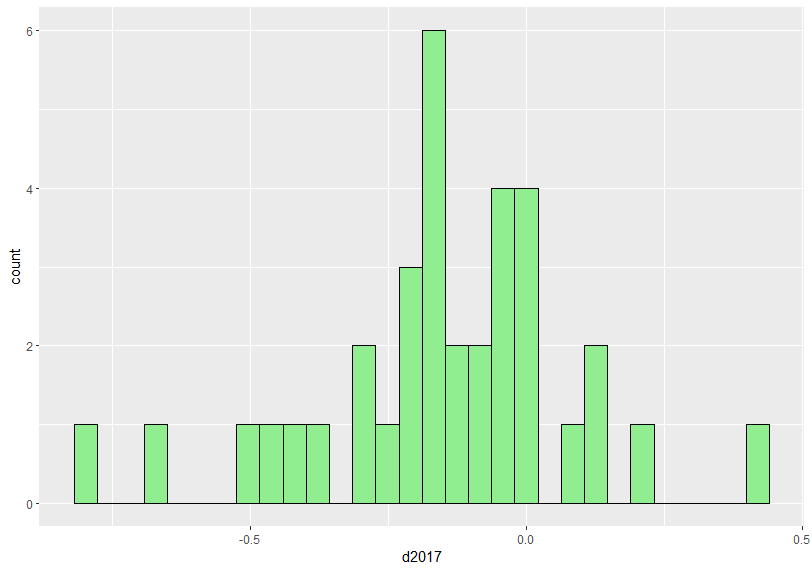The histogram of d2016 and the one of d2017 look very different.

I use t.test() function to check if there is siginificant difference between d2016 and d2017.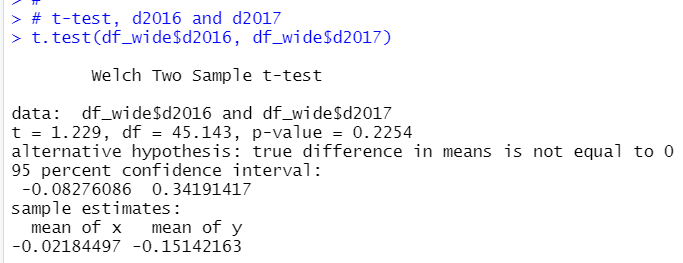p-value is 0.2254, greater than 0.05. So the both averages are not statistically different.

I use wilcox.test() function to see there is statistically difference of distribution.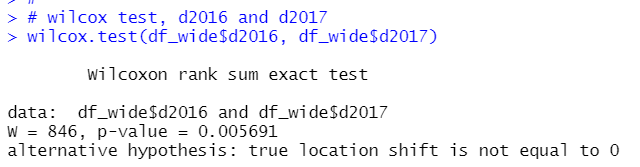p-value is 0.005691, less than 0.05. It means d2016 and d2017 locations are statistically different.

Let's make a scatter plot.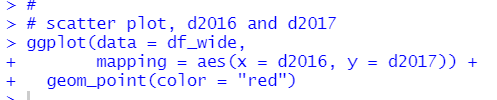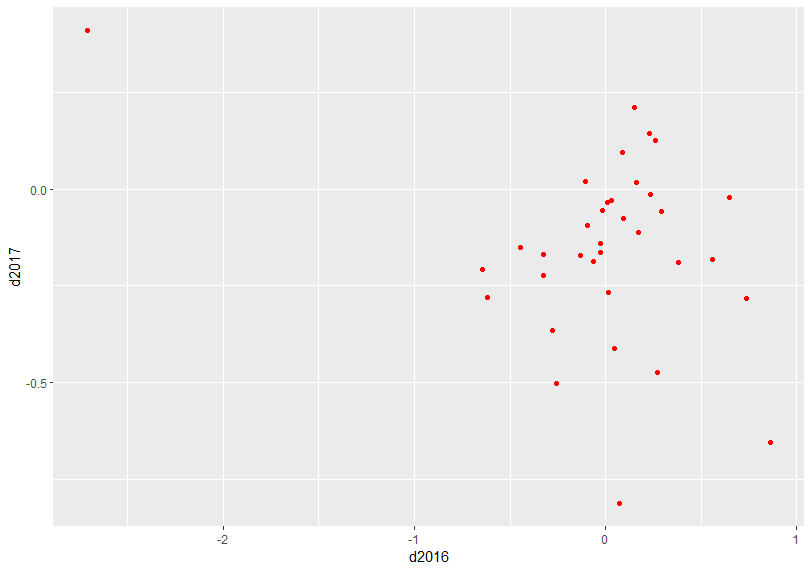Let's use cor.test to check there is statistically significant correlation between d2016 and d2017.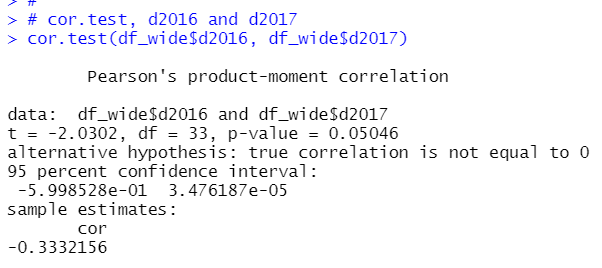p-value is 0.05046, slightly greater than 0.05. I can't say there is statistically correlation between d2016 and d2017.

Let's do Monte Carlo simulation.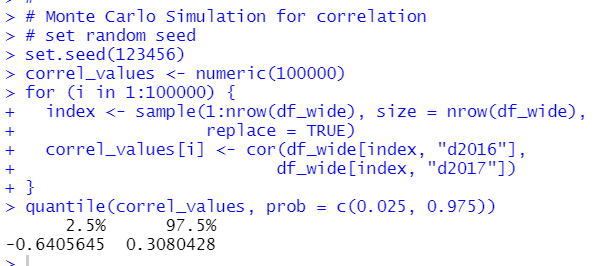95% confidence interval is from -0.64 to 0.308. So, there is not statistically positive/negative correlation.

That's it. Thank you!

The next post is